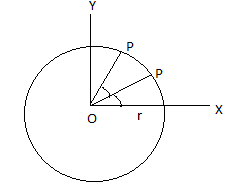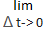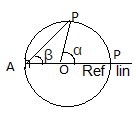Circular Motion for IIT JEE

Types of Circular Motion - Uniform and Non-Uniform Circular Motion

Introduction of Circular Motion

The motion of an object is said to be circular if it moves in a way that its distance from the center point always remains the same. When any object is roughly moving on a circular path it is called as circular motion. The speed of the body during the motion can either change or it may be the same throughout the whole motion. However, the velocity and direction of the motion will definitely change because its direction is changing at every point of the circle.

Here are some examples of circular motion – an artificial satellite orbiting the earth at a constant height; a stone which is tied to a rope and is being swung in circles, a car is turning through a curve on a race track.

Types of Circular Motion

There are two types of circular motion mentioned below:

• 1. Uniform circular motion

• 2. Non-uniform circular motion.

• Uniform Circular Motion
When the motion of an object in a circle is at a constant speed, it is called uniform circular motion. An object that moves in a circle is constantly changing its direction. This means that an object that is forming a circle on a path along with that particular object will complete repeated strips around the path in the same amount of period at every time. For instance, the object is moving at a tangent to the circle.

Below are some examples of uniform circular motion:

• a) The rotation of the blades of the ceiling fan.

• b) A racing car is turning through a curve on the racing track.

• c) A ball rolling on a floor with a constant velocity.

• d) A stone tied to a rope and being swung in circles.

• e) An artificial satellite orbiting the earth at a constant height.

• f) Different planets revolving at their own pace around the sun or around other planets.

• g) The hour hand of a clock.

• h) A pendulum having equal amplitudes on both the sides.

• i) A vibrating spring in a sewing machine.

• j) A rain drops falling at a uniform speed.

• k) A train going along the tracks at a constant speed.

• l) A ship streaming on a straight course at a constant speed.

• m) Electrons revolving around the nucleus, inside a atom, due to coulombic force.

• Non-Uniform Circular Motion – When a motion of an object denotes changes in the speed of the particle moving along the circular path, it is called non-uniform circular motion.

The changes that take place in the direction are accounted by radial acceleration which is given by the given equation.
ar = v2/r

Changes that take place in the speed have implications for radial acceleration. There may be two possibilities that are as follows:

• 1. The radius of the circle is constant just like in the motion along a circular rail or motor track. Changes that take place in v change the magnitude of the radial acceleration. This simply means that the centripetal acceleration is nonstop. The object moving at high speed will definitely need a greater radial which forces to change the direction and vice-versa when the radius of the circular path is constant.

• 2. In other possibility, the radial (centripetal) force is constant (like – a satellite rotating about the earth under the influence of a constant force of gravity). The circulation motion is fixed in its radius in regard to changes in speed. This simply means that unlike in the case of uniform motion, the radius of the circular path is variable. Eventually, the equation of the centripetal acceleration must be satisfied in terms of ‘speed’ and ‘radius’. However, the most important thing that is to be noted is that the change in speed of the particle affects the radial acceleration but the changes in speed is not affected by the radial or centripetal force.

• Below are the examples of non-uniform circular motion:

• a) A bouncing ball.

• b) A horse running in a race.

• c) A bus on its way through the market.

• d) Dragging a box from a path.

• e) Movement of an asteroid.

• f) Aircraft moving through the clouds and then landing.

• g) A train coming to its termination stop.

• h) A car coming to a halt.

• i) A car colliding with another car.

• j) A man running in 50m race.

• In the above mention examples, the speed is varying.

Kinematics of Circular Motion

Variable of
Motion

• a) Angular Position-

•The angle which is made by the position vector with the given line is called angular position. Two dimensional motion or motion in a plane is a circular motion.

For instance- Suppose a particle P is moving in a circle having radius r and centre O. The position of the object P at a given instant may be expressed by the angle q between OP and OX. This q angle is called angular position of the particle. As the object moves in the circle its angular position q change.

• b) Angular Displacement

• An angle rotated by a position vector of the moving object in the mentioned time interval with some reference line is called angular displacement.

Important points of angular displacement:

• • Angular displacement is dimensionless and has proper SI unit radian while other units are degrees or revolution 2p rad =3600 1 rev.

• • Finite angular displacement is not a vector quantity while infinity small angular displacement is a vector displacement because the addition of the small angular displacement is commutative while for large angular displacement it is not.

• • Direction of the small angular displacement is decided by ‘Right Hand Thumb Rule’. When the figures are conducted along the motion of the point then the thumb will show the direction of the angular displacement.

• • It can be different for various observers.

• c) Angular Velocity w

• i) Average angular velocity

• w = total angle of rotation/ total time taken

Therefore; θ2 – θ1 / t2 – t1 = Δ θ/ Δt

Where, θ1 & θ2 are angular position of the particle at time t1 & t2 respectively.

• ii) Instantaneous angular velocity

• The rate at which the position vector of a particle with respect to the centre rotates, it is called instantaneous angular velocity with respect to the centre.

w =Δ θ/ Δt = dθ/dt
Important points:
• Angular velocity is an axial vector with dimensions (T-1) and SI unit rad/s.

• Angular velocity is known as a characteristic of the body as a whole e.g. angular velocity of all points of earth about its own axis is (2p/24) rad/ hr, for a solid body as all points will rotate through the same angle in the same time.

• If a body takes ‘t’ seconds to make ‘n’ rotations then angular velocity in radian per second will be wav =2r/t

• Where, t = period and f = frequency of uniform circular motion
Wav = 2π x 1/T = 2πf

Where θ = a – bt + ct2 then w = /dt = -b + 2ct

• d) Relative Angular Velocity

• Angular velocity is described with respect of the point from which the position vector of the moving object is drawn. The angular velocity of the particle w.r.t ‘O’ and ‘A’ will be different here. It is mentioned as below:

WPO = /dt

Therefore, wPA = dβ/dtDynamic Circular Motion:

In two perpendicular directions, Newton’s law is applied in a circular motion. The first direction is along with the tangent and the other direction is perpendicular to it (towards the centre). The component of force acting along the tangent is called the tangential (Ft) and the component of force acting at the mid is called the centripetal force (Fc).

Tangential force Ft = M at = M dv/dt = Mαr
Centripetal force Fc = M at = mw2 r = m v2 /r
Note: If the centripetal force is absent, the object will move in a straight line with constant speed.
Instantaneous circle (R) has radius of:
R = [1+ (dy/dx)2]3/2/ |d2y/dx2|

Did You Know About These Basic Tricks?

• • Both centrifugal and centripetal forces are equal in magnitude and opposite in directions.

• • These both centripetal and centrifugal forces cannot be termed as action and reaction since action and reaction never act on the same body.

• • To complete the motion in a vertical circle is the basic requirement for a body under the limited conditions, For example, the stress in the string that must not vanish before it reaches the highest point. If it vanishes earlier, it will be devoid of the necessary centripetal force required to keep the body moving in a circle.

• • Centrifugal force is known as the fictitious force which acts on a body, rotating with a uniform velocity in a circle and along with the radius away from the centre.

• Hints You Need To Know